# 相似性和距离度量 (Similarity & Distance Measurement)

### 范叶亮 / 2019-01-01

$$d: X \times X \to \mathbb{R}$$

1. $d \left(x, y\right) \geq 0$ (非负性)
2. $d \left(x, y\right) = 0, \text{当且仅当} \ x = y$ (同一性)
3. $d \left(x, y\right) = d \left(y, x\right)$ (对称性)
4. $d \left(x, z\right) \leq d \left(x, y\right) + d \left(y, z\right)$ (三角不等式)

## 明可夫斯基距离 (明氏距离, Minkowski Distance)

$$d \left(x, y\right) = \left(\sum_{i=1}^{n} |x_i - y_i|^p\right)^{\frac{1}{p}}$$

$p = 1$ 时，称之为 曼哈顿距离 (Manhattan Distance)出租车距离

$$d \left(x, y\right) = \sum_{i=1}^{n} |x_i - y_i|$$

$p = 2$ 时，称之为 欧式距离 (Euclidean Distance)

$$d \left(x, y\right) = \sqrt{\sum_{i=1}^{n} \left(x_i - y_i\right)^2}$$$p \to \infty$ 时，称之为 切比雪夫距离 (Chebyshev Distance)

$$d \left(x, y\right) = \lim_{p \to \infty} \left(\sum_{i=1}^{n} |x_i - y_i|^p\right)^{\frac{1}{p}} = \max_{i=1}^{n} |x_i - y_i|$$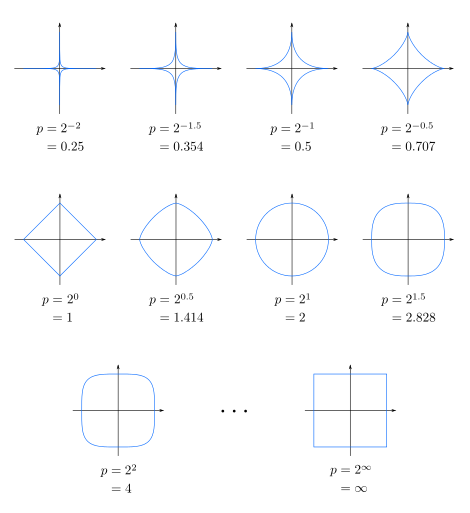## 马哈拉诺比斯距离 (马氏距离, Mahalanobis Distance)

$$d \left(x, y\right) = \sqrt{\left(x - y\right)^{\top} {\sum}^{-1} \left(x - y\right)}$$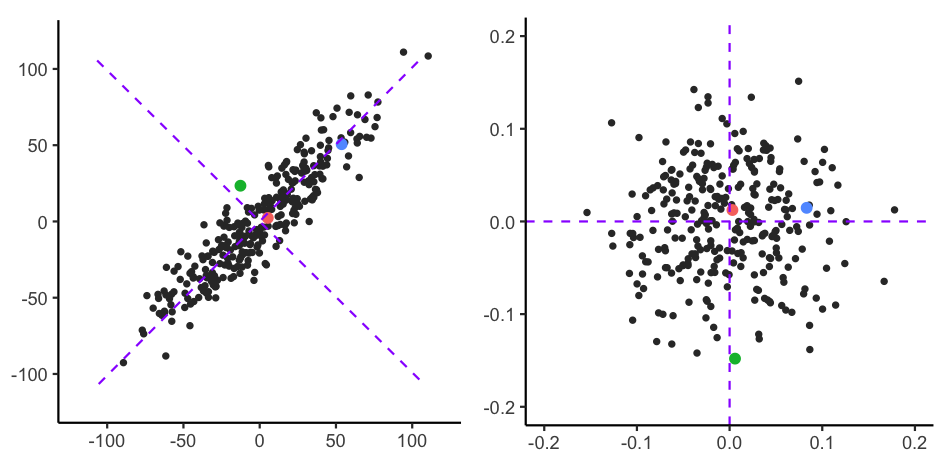## 向量内积 (Inner Product of Vectors)

$$x \cdot y = \sum_{i=1}^{n}{x_i y_i}$$

$$x \cdot y = \left| x \right| \left| y \right| \cos \theta$$

$$s \left(x, y\right) = \cos \left(\theta\right) = \dfrac{x \cdot y}{\left| x \right| \left| y \right|} = \dfrac{\sum_{i=1}^{n}{x_i y_i}}{\sqrt{\sum_{i=1}^{n}{x_i^2}} \sqrt{\sum_{i=1}^{n}{y_i^2}}}$$

$$\rho \left(x, y\right) = \dfrac{\text{cov} \left(x, y\right)}{\sigma_x \sigma_y} = \dfrac{E \left[\left(x - \mu_x\right) \left(y - \mu_y\right)\right]}{\sigma_x \sigma_y}$$

$$\begin{equation} \begin{split} r &= \dfrac{\sum_{i=1}^{n}{\left(x_i - \bar{x}\right) \left(y_i - \bar{y}\right)}}{\sqrt{\sum_{i=1}^{n}{\left(x_i - \bar{x}\right)^2}} \sqrt{\sum_{i=1}^{n}{\left(y_i - \bar{y}\right)^2}}} \\ &= \dfrac{1}{n-1} \sum_{i=1}^{n}{\left(\dfrac{x_i - \bar{x}}{\sigma_x}\right) \left(\dfrac{y_i - \bar{y}}{\sigma_y}\right)} \end{split} \end{equation}$$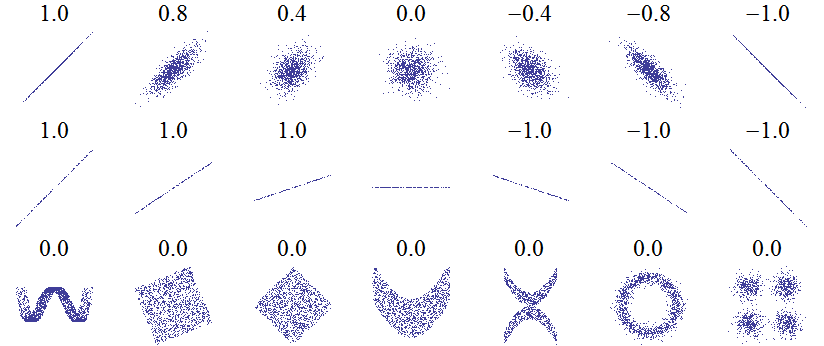## 集合距离 (Distance of Sets)

• Jaccard 系数

$$s = \dfrac{\left|X \cap Y\right|}{\left| X \cup Y \right|} = \dfrac{\left|X \cap Y\right|}{\left|X\right| + \left|Y\right| - \left|X \cap Y\right|}$$

Jaccard 系数的取值范围为：$\left[0, 1\right]$，0 表示两个集合没有重合，1 表示两个集合完全重合。

• Dice 系数

$$s = \dfrac{2 \left| X \cap Y \right|}{\left|X\right| + \left|Y\right|}$$

• Tversky 系数

$$s = \dfrac{\left| X \cap Y \right|}{\left| X \cap Y \right| + \alpha \left| X \setminus Y \right| + \beta \left| Y \setminus X \right|}$$

## 字符串距离 (Distance of Strings)

• Levenshtein 距离

Levenshtein 距离是 编辑距离 (Editor Distance) 的一种，指两个字串之间，由一个转成另一个所需的最少编辑操作次数。允许的编辑操作包括将一个字符替换成另一个字符，插入一个字符，删除一个字符。例如将 kitten 转成 sitting，转换过程如下：

$$\begin{equation*} \begin{split} \text{kitten} \to \text{sitten} \left(k \to s\right) \\ \text{sitten} \to \text{sittin} \left(e \to i\right) \\ \text{sittin} \to \text{sitting} \left(\ \to g\right) \end{split} \end{equation*}$$

• Jaro-Winkler 距离

$$sim = \begin{cases} 0 & \text{if} \ m = 0 \\ \dfrac{1}{3} \left(\dfrac{m}{\left|s_1\right|} + \dfrac{m}{\left|s_2\right|} + \dfrac{m-t}{m}\right) & \text{otherwise} \end{cases}$$

Jaro-Winkler 相似度给予了起始部分相同的字符串更高的分数，其定义为：

$$sim_w = sim_j + l p \left(1 - sim_j\right)$$

• 汉明距离

## 信息论距离 (Information Theory Distance)

$$P \left(X = x_i\right) = p_i, \quad i = 1, 2, ..., n$$

$$H \left(X\right) = - \sum_{i=1}^{n} P \left(x_i\right) \log P \left(x_i\right) \label{eq:entropy}$$

$$H \left(X\right) = - p \log p - \left(1-p\right) \log \left(1-p\right)$$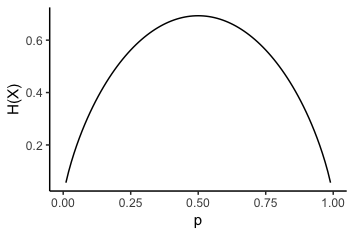$$P \left(X = x_i, Y = y_i\right) = p_{i, j}, \quad i = 1,2,...,n; \quad j = 1,2,...,m$$

$$\begin{equation} \begin{split} H \left(Y | X\right) &= \sum_{i=i}^{n} P \left(x_i\right) H \left(Y | X = x_i\right) \\ &= \sum_{i=1}^{n}{\sum_{j=1}^{m}{P \left(x_i, y_j\right) \log \dfrac{P \left(x_i\right)}{P \left(x_i, y_j\right)}}} \end{split} \end{equation}$$

$$H \left(X, Y\right) = \sum_{i=1}^{n}{\sum_{j=1}^{m}{P \left(x_i, y_j\right) \log P \left(x_i, y_j\right)}}$$

• 互信息 (Mutual Information)

$$I \left(X; Y\right) = \sum_{i=1}^{n}{\sum_{j=1}^{m}{P \left(x_i, y_j\right) \log \dfrac{P \left(x_i, y_i\right)}{P \left(x_i\right) P \left(y_j\right)}}}$$

• 相对熵 (Relative Entropy)

$$D_{KL} \left(P \| Q\right) = \sum_{i}{P \left(i\right) \ln \dfrac{P \left(i\right)}{Q \left(i\right)}}$$

KL 散度为非负数 $D_{KL} \left(P \| Q\right) \geq 0$，同时其不具有对称性 $D_{KL} \left(P \| Q\right) \neq D_{KL} \left(Q \| P\right)$，也不满足距离函数的三角不等式。

$$\begin{equation} \begin{split} H \left(P, Q\right) &= H \left(P\right) + D_{KL} \left(P \| Q\right) \\ &= - \sum_{i}{P \left(i\right) \log Q \left(i\right)} \end{split} \end{equation}$$

• JS 散度 (Jensen-Shannon Divergence)

JS 散度解决了 KL 散度不对称的问题，定义为：

$$D_{JS} \left(P \| Q\right) = \dfrac{1}{2} D_{KL} \left(P \| \dfrac{P + Q}{2}\right) + \dfrac{1}{2} D_{KL} \left(Q \| \dfrac{P + Q}{2}\right)$$

• 推土机距离 (Earth Mover Distance, Wasserstein Distance)

1. 运送需从 $p_i$$q_j$，不能反向，即 $f_{i, j} \geq 0, 1 \leq i \leq m, 1 \leq j \leq n$
2. $p_i$ 运送出的总重量不超过原始的总重量 $w_{pi}$，即 $\sum_{j=1}^{n}{f_{i, j}} \leq w_{pi}, 1 \leq i \leq m$
3. 运送到 $q_j$ 的总重量不超过其总容量 $w_{qj}$，即 $\sum_{i=1}^{m}{f_{i, j}} \leq w_{qj}, 1 \leq j \leq n$
4. $\sum_{i=1}^{m}{\sum_{j=1}^{n}{f_{i, j}}} = \min \left\{\sum_{i=1}^{m}{w_{pi}}, \sum_{j=1}^{n}{w_{qj}}\right\}$

$$\min \sum_{i=1}^{m}{\sum_{j=1}^{n}{d_{i, j} f_{i, j}}}$$

$$D_{W} \left(P, Q\right) = \dfrac{\sum_{i=1}^{m}{\sum_{j=1}^{n}{d_{i, j} f_{i, j}^*}}}{\sum_{i=1}^{m}{\sum_{j=1}^{n}{f_{i, j}^*}}}$$

## 其他距离 (Other Distance)

• DTW (Dynamic Time Warping) 距离

DTW 距离用于衡量两个序列之间的相似性，序列的长度可能相等也可能不相等。对于两个给定的序列 $X = \left(x_1, x_2, \cdots, x_m\right)$$Y = \left(y_1, y_2, \cdots, y_n\right)$，我们可以利用动态规划的方法求解 DTW 距离。首先我们构造一个 $m \times n$ 的矩阵，矩阵中的元素 $d_{i, j}$ 表示 $x_i$$y_j$ 之间的距离。我们需要找到一条通过该矩阵的路径 $W = \left(w_1, w_2, \cdots, w_l\right)$, $\max\left(m, n\right) \leq l < m + n + 1$，假设 $w_k$ 对应的矩阵元素为 $\left(i, j\right)$，对应的距离为 $d_k$，则 DTW 的优化目标为 $\min \sum_{k=1}^{l}{d_k}$。如下图右上角部分所示：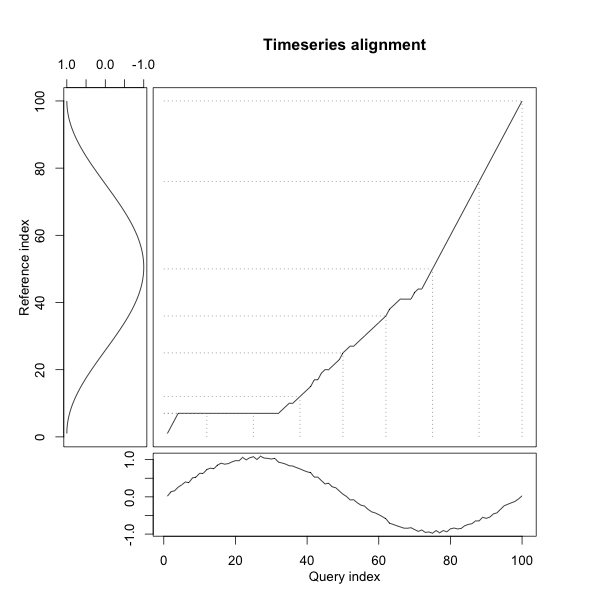1. 边界条件$w_1 = \left(1, 1\right), w_k = \left(m, n\right)$，即路径须从左下角出发，在右上角终止。
2. 连续性：对于 $w_{l-1} = \left(i', j'\right), w_l = \left(i, j\right)$，需满足 $i - i' \leq 1, j - j' \leq 1$，即路径不能跨过任何一点进行匹配。
3. 单调性：对于 $w_{l-1} = \left(i', j'\right), w_l = \left(i, j\right)$，需满足 $0 \leq i - i', 0 \leq j - j'$，即路径上的点需单调递增，不能回退进行匹配。

$$dtw_{i, j} = \begin{cases} 0 & \text{if} \ i = j = 0 \\ \infty & \text{if} \ i = 0 \ \text{or} \ j = 0 \\ d_{i, j} + \min \left(dtw_{i-1, j}, dtw_{i-1, j-1}, dtw_{i, j-1}\right) & \text{otherwise} \end{cases}$$

$dtw_{m, n}$ 则为最终的 DTW 距离。在 DTW 求解的过程中还可以使用不同的 Local Warping Step 和窗口类型，更多详细信息可看见 R 中 dtw 包。下图展示了利用 DTW 求解后不同点之间的对应关系：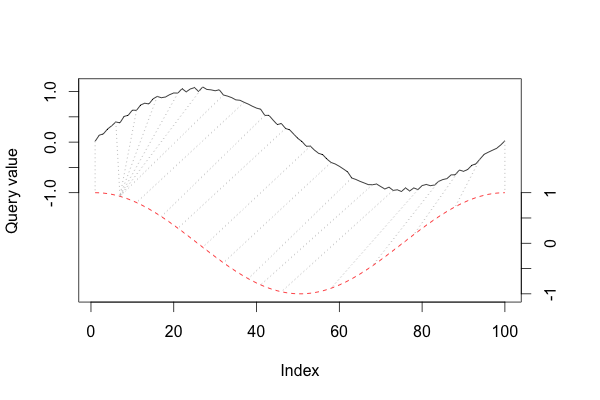• 流形距离 (Distance of Manifold)